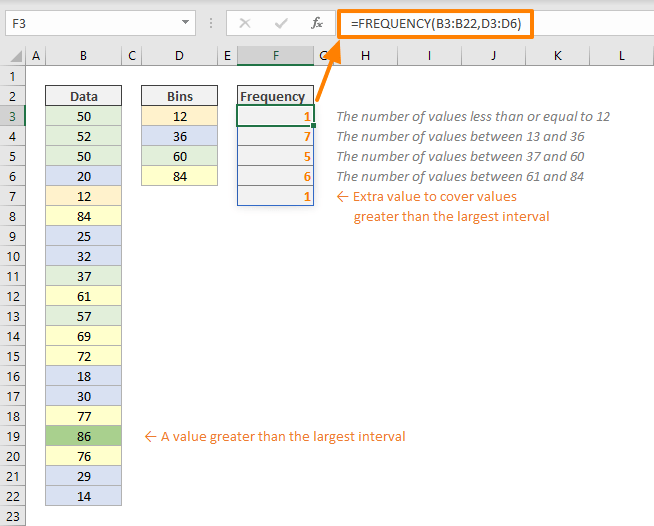FREQUENCY is a Statistical function that can calculate how often values occur in a set of data and returns the occurrence counts as a vertical array. These numbers represent frequencies can be helpful for charting histograms. In this guide, we’re going to show you how to use the FREQUENCY function and also go over some tips and error handling methods.

• All versions

## Syntax

FREQUENCY(data_array, bins_array)

Since the FREQUENCY function returns an array of numbers, the function can populate multiple cells in vertical direction. If you are a Microsoft 365 subscriber, simply entering formula will fill the cells. Otherwise, you need to enter the formula as an array formula.

Select the output range, enter the formula and press Ctrl + Shift + Enter to submit.

## Arguments of FREQUENCY Function

 data_array An array of values for which you want to count frequencies. bins_array An array of intervals into which you want to group the values in data_array.

## Examples

You need two arrays of numbers to use the FREQUENCY function. The first array (data_array) should contain the actual values. The second array (bins_data), on the other hand, needs to contain the values that determine the intervals. Each value represents the high limit of a bin, starting with the next lower value.

For example, if the bins_array has the numbers of 12, 36, 60 and 84, the intervals are the following:

• less than or equal to 12
• 13 - 36
• 37 - 60
• 61 - 84
• greater than 84

Although, the bins_array has 4 numbers, the FREQUENCY function generates 1 extra row to cover any value greater than the last interval.=FREQUENCY(B3:B22,D3:D6)

## Tips and Remarks

• The FREQUENCY function returns an array. Use it as an array formula if you are not using Microsoft 365
• Each bin shows a count of values up to, and including bin value, excluding the values already counted.
• The FREQUENCY function returns an extra value to catch any values greater than the largest interval.
• If data_array contains no values, FREQUENCY returns an array of zeros.
• If bins_array contains no values, FREQUENCY returns the number of elements in data_array.
• The FREQUENCY function ignores blank cells and text fields.
• Check the How to create an Excel histogram article to see a use case of the function.